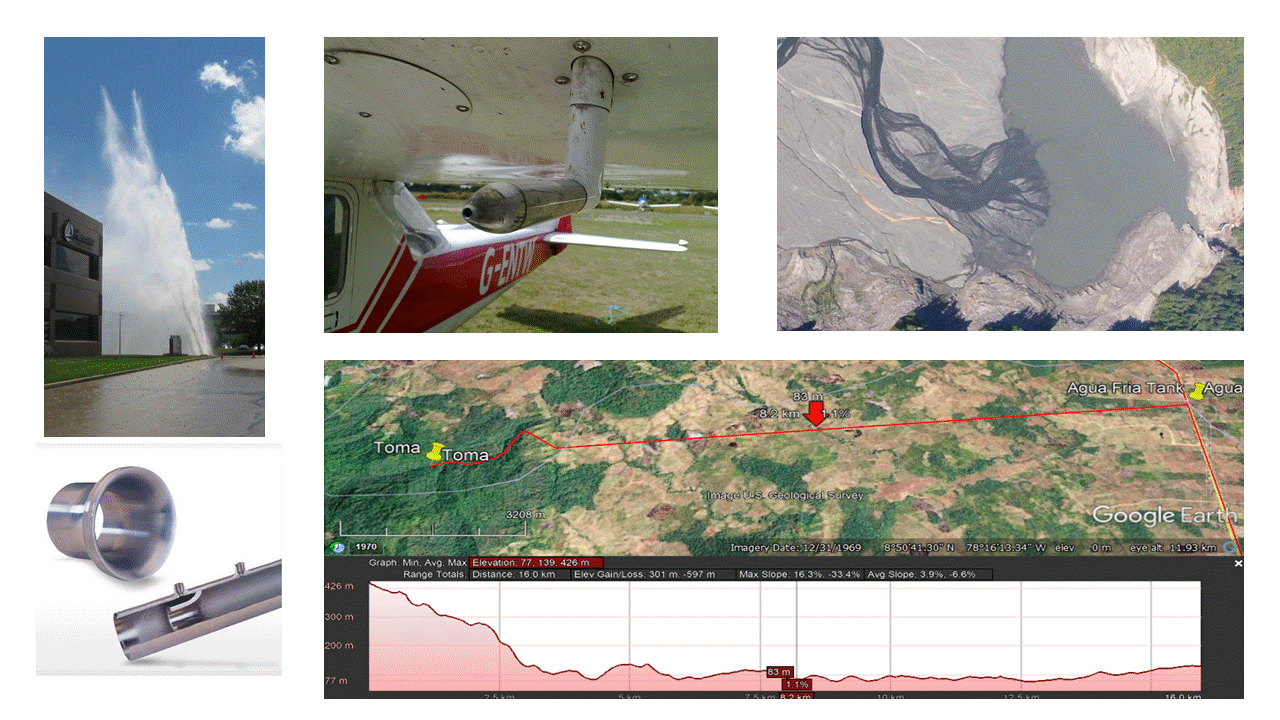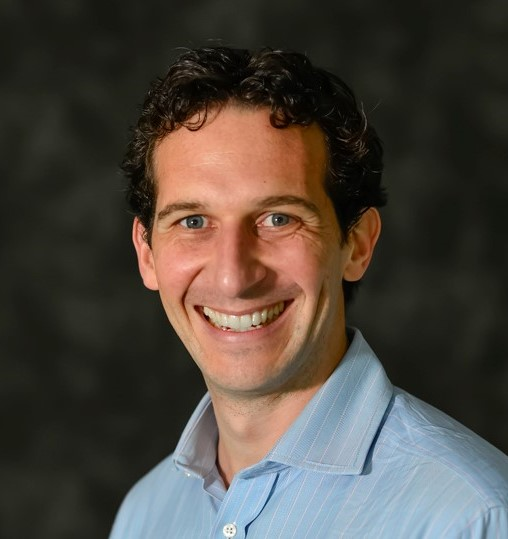Skip to main content

# Fluid Mechanics: Bernoulli's Equation

HydroLearn## Problem Statement

This module forms part of a larger series that examine the various aspects of the General Energy Equation. This particular module deals specifically with Bernoulli's Equation, which is a subset of the General Energy Equation.

The module starts by leading the student through the development of Bernoulli's Equation. Then Bernoulli's Equation is combined with the Continuity Equation and the Q-A-V relationship to allow students to show students extensions of Bernoulli's Equation within the practice of engineering. Students are then introduced to Hydraulic and Energy Gradelines and piezometric head. To culminate, students are led through applications of Bernoulli's Equation.

Each of the four sections contains calculations to help students understand concepts and apply Bernoulli's Equation with a final learning activity designed to challenge students at higher cognitive levels. The final activity involves having students create a video that demonstrates their understanding of Bernoulli's Equation and includes a student-designed demonstration of Bernoulli Equation principles.

## Module Overview

This module forms part of a larger series of modules that examine the various aspects of the General Energy Equation. This particular module deals specifically with Bernoulli's Equation, which is a subset of the General Energy Equation.

## Topics Covered

Development of the Bernoulli's Equation; Combining the Continuity and Bernoulli's equations; Hydraulic and Energy Grade Lines; Bernoulli's Equation Applications

## Prerequisites

This module is part of a series of topics in basic fluid mechanics. Required knowledge includes fluid properties (density, specific weight, viscosity, pressure, among others) and fluid statics.

## Learning Objectives

1. Given a control volume with a single inlet and a single outlet, students will be able to write Bernoulli’s equation for the flow of a liquid (head form) from memory [Remember];

2. Students will be able to distinguish the key assumptions governing the use of Bernoulli’s equation and their interpretations when applied to a real-world problem [Understanding];

3. Students will be able to convert between the pressure and head form of Bernoulli’s equation [Understand];

4. Students will be able to apply Bernoulli’s equation to relate pressure and velocity to compute lift force for flat surfaces [Apply];

5. For inviscid flow, students will be able to solve for flow rate in a system using Bernoulli’s equation in conjunction with the continuity equation for an obstruction flow meter [Apply];

6. For incompressible fluid flow, students will be able to relate the volumetric flow rate, area, and velocity of flow between two points in a piping system using the continuity equation [Apply];

7. Students will be able to evaluate a piping system by plotting the hydraulic grade line (HGL) and energy grade line (EGL) to determine required pressure breaks [Evaluate];

8. Given inviscid conditions, students will be able to solve for an unknown property value (pressure, velocity, or elevation) using Bernoulli’s equation along a streamline [Apply];

9. Students will be able to produce a video of an application of Bernoulli’s equation in the context of a commonplace situation from their own experience [Create];

10. Students will be able to communicate the results of their technical assessments in a professional and creative format [ABET Communication].

This will be accomplished through activities within each section. Results from each activity will be recorded in specified results templates. The results templates for each activity can be found at the beginning of each activity. The results templates are organized such that results from one activity can easily be used in successive activities.

## Course Authors### William S. Gonwa

William Gonwa has worked in the fields of wastewater collection, storm water management, and flood protection since 1984. He has received degrees from Marquette University, the University of Kentucky, and the University of Wisconsin-Madison, all in civil and environmental engineering. After practicing consulting engineering for 25 years, Dr. Gonwa joined the Milwaukee School of Engineering full-time faculty in 2010 where he is now an associate professor and program director for Civil Engineering (Contact).### Mauricio E. Arias

Mauricio Arias is an Assistant Professor in the Department of Civil and Environmental Engineering at the University of South Florida (Tampa), where he is the Principal Investigator of the Watershed Sustainability Lab and teach water resources undergraduate and graduate courses. He holds a Bachelors and a Masters in Environmental Engineering Sciences from the University of Florida, and a PhD in Civil Engineering from the University of Canterbury (New Zealand). He returned to Florida in 2016 after completing a Post-doctoral research fellowship in Sustainability Science at Harvard University (Contact).

## Target Audience

The target audience is sophomore or junior students in an introductory fluid mechanics course.

## Tools Needed

Students should have access to the Internet and basic video production equipment such as a cell phone and basic video-editing software. Microsoft Excel and Google EarthPro are also needed for two of the learning activities. Students will also need a printer or electronic means to compile and submit assignments.)

## Expected Effort

It is estimated that students will take 8 to 12 hours to complete this module including all learning activities

1. Course Export

Export Link
2. Course Number

HL300
3. Estimated Effort

12:00
Enroll